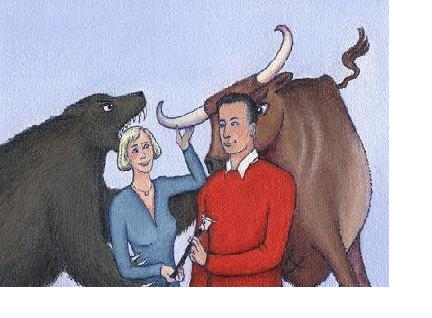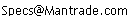Daily Speculations The Web Site of Victor Niederhoffer & Laurel Kenner Dedicated to the scientific method, free markets, deflating ballyhoo, creating value, and laughter;  a forum for us to use our meager abilities to make the world of specinvestments a better place.

Write to us at:(address is not clickable)

As a starting point, we draw monthly adjusted close data from Yahoo! Finance, calculate returns and compare that to the S&P500 returns (after subtracting the risk free rate from both), using least-squares linear regression. Next, we perform the same calculation using robust regression (from the WLE package) which is resistant to outliers and leverage points. Then, the function downloads monthly data made available by Kenneth R. French on his website, namely his "Fama/French Factors". These include SMB, HML, the Market, and the risk free rate. The HML factor is the returns of high book to market (value) stocks relative to low book to market (growth) companies Some also think of HML as a proxy for "distress" or related risk factor. Factor SMB is the returns of small size (market cap) stocks relative to large ones, which some also consider a proxy for risk related to liquidity or maturity.

Next, we calculate a Fama/French three factor CAPM ("FF model"), using robust multiple regression, with company return (minus the risk free rate) being explained by 1)market returns minus the risk free rate, 2) SMB (size), and 3)HML (value).

The function calculates and outputs the following:

 "CAPM beta= 0.394964815720561"

 "robust stock beta= 0.195777679517487"

 "robust ff beta= 0.564194766674277"

 "robust ff smb= -0.246388978409093"

 "robust ff hml= 0.759689811530004"

 "regular coe= 6.9748240786028 robustcoe= 5.97888839758744"

 "Fama-French coe= 9.0939644780223"

 "FF superior to CAPM"

which is:

CAPM Beta coefficient

CAPM Beta using robust regression

Market Beta in a robust FF-3-factor model

SMB coefficient in a robust FF-3-factor model

HML coefficient in a robust FF-3-factor model

COE under CAPM and robust CAPM(assuming 5% market risk premium and 5% riskfree rate)

COE under FF-3-factor model (assuming 5% market risk premium, 5% riskfree rate, 1% return premium for smallcap stocks, and 2% return premium for value stocks)

The final statement declares which model is superior statistically, measured by Akaike's An Information Criterion (AIC), a more stringent model comparison test than say, Adjusted R-squared.

Two graphs also pop up. On top is a scatterplot matrix showing actual stock returns versus the returns fitted by the FF-3factor model (left middle panel), stock returns versus fits for the CAPM model (bottom left panel), and FF-3factor fits versus CAPM model fits. The top center panel displays the correlation between stock returns and the FF-model, while the top right panel displays the correlation between stock returns and the CAPM model.

The second chart, underneath the top chart, shows the CAPM regression line, with the robust CAPM regression line drawn in red for contrast, illustrating to what extent outliers and/or influence points may have effected the CAPM fit.

For most companies we queried, the FF-3-factor model provided a statistically superior explanation of returns. In most cases, the resulting COE estimate also provided a more "reasonable" figure than that provided by the CAPM model.

Here is an example:

(Assume you have loaded R, have installed the "MASS", "tseries", "zoo", and "wle" packages from CRAN, and have internet access enabled. Then you simply need to copy and paste in the function code, hit return, and type the following, followed by a return )

getffBeta("DUK")

Inspecting the top graph - note that the FF-model provides a much tighter fit to actual returns. Correlation between fits is twice that of the CAPM model.

Inspecting the second graph, one notes that DUK's CAPM fit is particularly weak, it was particularly influenced by outliers/influence points.

 "CAPM beta= 0.394964815720561"

 "robust stock beta= 0.195777679517487"

 "robust ff beta= 0.564194766674277"

 "robust ff smb= -0.246388978409093"

 "robust ff hml= 0.759689811530004"

 "regular coe= 6.9748240786028 robustcoe= 5.97888839758744"

 "Fama-French coe= 9.0939644780223"

 "FF superior to CAPM"

Note that in the FF-3-factor context, market beta rises, and the HML (value) factor is quite significant, as one might expect for a utility. Being a large company, DUK has a negative loading on the SMB (size) factor. Overall, the 9% COE estimate makes more sense than a 5% to 6% estimate.

COE calculation: 5%(rf) + .395(mkt beta)*5%(mkt risk prem) = 6.97%

CAPM calculation 5%(rf) + .56(mkt beta)*5%(mkt risk prem) -.246(SMB beta)*(1% size premium)+ .76(HML beta)*(2% value premium).

Note that the Fama French model does not always increase a cost of equity estimate. Let's examine a counter-example in technology: Sun Microsystems (SUNW)

getffBeta("SUNW")

 "CAPM beta= 2.21214087925452"

 "robust stock beta= 2.20953948669588"

 "robust ff beta= 1.79969580208857"

 "robust ff smb= -0.0091490620014999"

 "robust ff hml= -1.04954100605994"

 "regular coe= 16.0607043962726 robustcoe= 16.0476974334794"

 "Fama-French coe= 11.8902479363215"

 "FF superior to CAPM"

Above, regular CAPM overestimates market Beta, as well as COE, because it is omits a significant negative coefficient to HML, (though size (SMB) is not significant). Regular CAPM suggests an unreasonable COE of 16%. The Fama-French model suggests a more reasonable 11.9% COE, due to lower estimated market beta, and a negative coefficient to the HML factor.

CAPM COE: 5% + 2.21*5% = 16%

FF COE : 5% +1.8*5% -1.05*2%= 11.9%

Conclusion:

We have provided a couple of examples for which the Fama-French three factor model provides a superior explanation of past returns and more reasonable Cost of Equity estimates. Users of R with an interest in the subject are encouraged to take a look at companies or funds with which they are familiar.

Appendix:

The function: (not well annotated) - copy and paste into the R console.

getffBeta=function(tool) {

require(MASS);

require(tseries);

require(wle)

require(zoo)

stock= (get.hist.quote(instrument = tool, quote =c("Ad"),compression="m",origin= as.Date(0)));

spx=(get.hist.quote(instrument = "^gspc", quote =c("Ad"),compression="m",origin= as.Date(0)));

today=Sys.Date();

seqmo=length(seq(as.Date("1926-07-01"),today,by="month"))-2;

url="http://mba.tuck.dartmouth.edu/pages/faculty/ken.french/ftp/F-F_Research_Data_Factors.zip";

destfile=tempfile();

unzip=unz(destfile,"F-F_Research_Data_Factors.txt");

ffdata <- ff4

attach(ffdata);

ffdata=ffdata/100

#find out starting year month for ffdata;

ffdatats=ts(ffdata, start=c(1926,7), frequency=12);

stock=ts(na.omit(coredata(stock)), start= as.numeric(as.yearmon(as.Date(start(stock)))),

frequency=12);

spx=ts(na.remove(spx), start=

as.numeric(as.yearmon(as.Date(start(spx)))),

frequency=12);

combined= na.remove(ts.union(stock,spx));

combreturn= na.remove(diff(log(combined)));

combined=na.remove(ts.intersect(combreturn,ffdatats), names=list("stockret", "spxret", "dates","ffmktret","smb","hml","rf"));

combined[,1]=combined[,1]-combined[,7]

combined[,2]=combined[,2]-combined[,7]

simplereg=lm(combined[,1]~combined[,2]);

stockbeta=simplereg\$coef;

textout=paste("CAPM beta=", stockbeta);

robustreg=wle.lm(combined[,1]~combined[,2]);

robstockbeta=robustreg\$coef;

textout2=paste("robust stock beta=", robstockbeta);

plot(as.numeric(combined[,1])~as.numeric(combined[,2]),xlab="S&P",

ylab=tool)

title(main=textout, sub=list(textout2, col="red"))

abline(coef(simplereg));

abline(coef(robustreg),col="red")

print(textout);

print(textout2);

ffreg=wle.lm(combined[,1]~combined[,4]+combined[,5]+combined[,6]);

ffbeta=ffreg\$coef;

ffsmb=ffreg\$coef;

ffhml=ffreg\$coef ;

textout3=paste('robust ff beta=',ffbeta) ;

textout4=paste('robust ff smb=',ffsmb) ;

textout5=paste('robust ff hml=',ffhml) ;

print(textout3) ;

print(textout4) ;

print(textout5) ;

rf=5

smb=1

hml=2

rm=5

ffcoe=rf+ffbeta*rm+ffsmb*smb+ffhml*hml

robcoe=rf+robstockbeta*rm

regcoe=rf+stockbeta*rm

textout6=paste('regular coe=',regcoe,' robustcoe=',

robcoe)

textout7=paste('Fama-French coe=',ffcoe)

print(textout6)

print(textout7) ;

ffreg2=lm(combined[,1]~combined[,4]+combined[,5]+combined[,6]);

a1=as.vector(combined[,1])

b1=as.vector(ffreg2\$fitted)

c1=as.vector(simplereg\$fitted)

plot3= (data.frame(a1,b1,c1))

win.graph()

panel.cor <- function(x, y, digits=2, prefix="", cex.cor)

{

usr <- par("usr"); on.exit(par(usr))

par(usr = c(0, 1, 0, 1))

r <- abs(cor(x, y))

txt <- format(c(r, 0.123456789), digits=digits)

txt <- paste(prefix, txt, sep="")

if(missing(cex.cor)) cex <- 0.8/strwidth(txt)

text(0.5, 0.5, txt, cex = cex * r)

}

pairs(plot3, upper.panel=panel.cor,labels=c("stock returns","FF fits","CAPM fits"))

ffreg2=lm(combined[,1]~combined[,4]+combined[,5]+combined[,6]);

if((AIC(simplereg))<(AIC(ffreg2))) "CAPM superior to FF" else "FF superior to CAPM";

}